# Adjustable constant current source (100-500mA output)

#### kiweered

Joined Dec 13, 2021
25
Hello,

I need to drive small valves (you can see them as resistors which heats up and deforms to let the liquid flow). They usually need 250mA to be oppened but from my early testing it's sometime more sometime less.
I would like to design an adjustable by potentiometer constant current source to drive them.

I have acces to a 24V and 5V power supply but if possible I would like to only use 5V.
The output current of the current driver should be adjustable between [100mA -500mA].
It would be better to not have the potentiommeter in the 250mA current path for power dissipation issues.

I have looked at many options :
1) Linear Regulator current source :
Probably the easiest option but not sure if it's compatible here.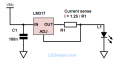The main issue here is that the potentiommeter R1 sees the entire output current so I checked this improved version :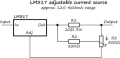But from what I understood you need at least 5.5V as input voltage (I didn't undestand why if you can explain) so I should use my 24V power supply but I'm afraid that the power dissipation would be far to big for a linear regulator (P=(Vin-Vout)*Iout). It's hard to measure the load but the voltage drop across the valve was 0.7V when 250mA current is going through.

2) OpAmp current source
By adjusting Vin with a voltage divider between 0-4V, and with R=10 instead of 1ohm, I shoud get Iout=[0-400mA].I simulated this with V+=5V and I found that Vin must be less than 1V in order to acheive VIN+=VIN- and I don't know why. And I think it's not PCB friendly to have 0,1V tensions. I put RL=4ohm (the resistance measured across the valve when it's not powered).

I also looked at transitor mirrors but same there, if you want to adjust the outputcurrent your potentiommeter will see the same current (a lot of heat).

If you have any experience with Linear Regulator current source can you say if it is possible ? I didn't acheive it with simultions.
What should I do for with the OpAmp circuit ? Change references ? Use 24V supply instead of 5 (I works on simulations but it seems so overkilled to use 24V for such aplication) ?
Any other circuit I could do ?

#### wayneh

Joined Sep 9, 2010
17,405
I'd pursue the op-amp approach. I built a variable, constant current source that way and it works great. Mine is 0-1mA and so it doesn't need the external transistor, but otherwise it's the same concept.

#### crutschow

Joined Mar 14, 2008
32,903
I found that Vin must be less than 1V in order to acheive VIN+=VIN-
The answer is on the schematic: Iout = Vin/1Ω, so the input voltage for 100-500mA would be 100-500mV.
If you want a larger signal at the input then use a resistive attenuator there.
Thus a 10:1 attenuator would then require an input signal of 1-5V for 100-500mA.

#### kiweered

Joined Dec 13, 2021
25
The answer is on the schematic: Iout = Vin/1Ω, so the input voltage for 100-500mA would be 100-500mV.
If you want a larger signal at the input then use a resistive attenuator there.
Thus a 10:1 attenuator would then require an input signal of 1-5V for 100-500mA.
This is what i've done :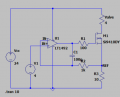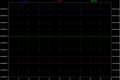With 24V supply this is working fine but with 5V supply it's not working :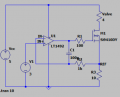You can check that I(valve)=215mA and not 300mA. If you increase VIN+ it will remain 215mA.
But you can compute 4R*0.3A+10R*0.3A=4.2V<5V.
Is it because of the Rail-to-Rail opAmp caracteristic ?

#### AnalogKid

Joined Aug 1, 2013
10,576
You show the valve as a constant 4 ohm resistance. If so, then it shouldn't matter if you drive it with a current source or voltage source. Either one will regulate the power to the valve. I don't see any advantage to the extra complexity of a constant current source. Varying the current by +/-40 mA would have the same effect as varying the voltage by +/-160 mV - both are 10% changes.

Wouldn't an adjustable 3-terminal LDO work?

https://www.analog.com/media/en/technical-documentation/data-sheets/1086ffs.pdf

ak

Last edited:
•crutschow

#### crutschow

Joined Mar 14, 2008
32,903
Is it because of the Rail-to-Rail opAmp caracteristic ?
No, it's likely that the MOSFET Vgs is the limit.
Its maximum Vgs(th) is 3V, thus the maximum voltage across R3 would be 2V, giving a maximum current of 200mA.
If you want it to work from 5V, then you need to reduce the value of R3.
For 500mA, that would be <4Ω.

#### kiweered

Joined Dec 13, 2021
25
You show the valve as a constant 4 ohm resistance. If so, then it shouldn't matter if you drive it with a current source or voltage source. Either one will regulate the power to the valve. I don't see any advantage to the extra complexity of a constant current source. Varying the current by +/-40 mA would have the same effect as varying the voltage by +/-160 mV - both are 10% changes.

Wouldn't an adjustable 3-terminal LDO work?

https://www.analog.com/media/en/technical-documentation/data-sheets/1086ffs.pdf

ak
I think that's the problem, so far I'm driving these valves with a simple low side mosfet switch and a set the current with a resistor but the behaviour is not constant. I feel like these small valves are kind of random in their specs so that's why I think an adjustable current drive might work better.

No, it's likely that the MOSFET Vgs is the limit.
Its maximum Vgs(th) is 3V, thus the maximum voltage across R3 would be 2V, giving a maximum current of 200mA.
If you want it to work from 5V, then you need to reduce the value of R3.
For 500mA, that would be <4Ω.
I'm sorry I don't understand. Can you explain more in detail please?
You want the voltage across R3 to be higher than Vgs(th) or lower ? And if lower why ? I thought in order to "open" the Vgs should be higher than the threshold voltage.
And if you have 5 or 24V the voltage across R3 is the same no ?

#### AnalogKid

Joined Aug 1, 2013
10,576
Valve part number / data sheet / link - ?

ak

#### crutschow

Joined Mar 14, 2008
32,903
You want the voltage across R3 to be higher than Vgs(th) or lower ?
It has nothing directly to do with it being higher or lower.
The answer to that question is, it can be either, depending upon circuit parameters including supply voltage.
I thought in order to "open" the Vgs should be higher than the threshold voltage.
Yes,
But in electronics "open" means not conducting (as in saying it's an "open circuit").
"Conduct" is a better term.
And if you have 5 or 24V the voltage across R3 is the same no ?
Only if it stays in the linear region.
You always need to determine if that's true in analyzing analog circuit operation.
The supply voltage, which here equals the maximum gate voltage from the opamp, thus determines the maximum voltage across R3, above which the circuit operation is no longer linear.

Thus for a 5V supply, the maximum Vg is 5V, so Vs (MOSFET maximum source voltage) is 5V-Vgs(th) = 2V, thus the maximum current is 2V / R3.
So for 500mA maximum, the value of R3 can not be more than 2V / 500ma = 4Ω for the circuit to stay in the linear mode.

Make sense now?

#### kiweered

Joined Dec 13, 2021
25
It has nothing directly to do with it being higher or lower.
The answer to that question is, it can be either, depending upon circuit parameters including supply voltage.
Yes,
But in electronics "open" means not conducting (as in saying it's an "open circuit").
"Conduct" is a better term.
Only if it stays in the linear region.
You always need to determine if that's true in analyzing analog circuit operation.
The supply voltage, which here equals the maximum gate voltage from the opamp, thus determines the maximum voltage across R3, above which the circuit operation is no longer linear.

Thus for a 5V supply, the maximum Vg is 5V, so Vs (MOSFET maximum source voltage) is 5V-Vgs(th) = 2V, thus the maximum current is 2V / R3.
So for 500mA maximum, the value of R3 can not be more than 2V / 500ma = 4Ω for the circuit to stay in the linear mode.

Make sense now?
Yes now I get it, I forgot that the opamp output is linear between -+VCC. So if I chose an other NMOS with a lower threshold voltage I could get a higher voltage range across R3?

#### crutschow

Joined Mar 14, 2008
32,903
I forgot that the opamp output is linear between -+VCC.
Only if the loop is not saturated, as when the op amp output tries to go above its supply voltage.
So if I chose an other NMOS with a lower threshold voltage I could get a higher voltage range across R3?
Yes, but wouldn't it be easier just to reduce the value of R3?
Alternately, you could use an NPN BJT which has a drop of only about 0.7V between base and emitter.